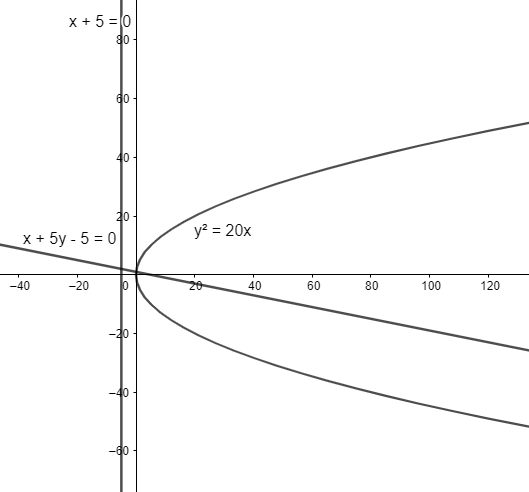QuestionAnswers

# The circle drawn with variable chord $x+ay-5=0$($a$being parameter) of the parabola${{y}^{2}}=20x$as diameter will always touch the line(a) $x+5=0$(b) $y+5=0$(c) $x+y+5=0$(d) $x-y+5=0$Hint: To find the circle with variable chord of the parabola as its diameter, we will
first find the exact equation of the chord and then use the fact that a circle drawn with a
chord to the parabola as its diameter has a tangent which is directrix to the parabola.We
have a variable chord $x+ay-5=0$ of the parabola${{y}^{2}}=20x$.

We want to find the equation of tangent to the circle with the variable chord of the parabola
as its diameter.
We will consider the parabola${{y}^{2}}=4ax$.
By comparing it with our parabola${{y}^{2}}=20x=4\times 5x$, we observe that$a=5$.Substituting the value of$a$in the equation of the chord$x+ay-5=0$of the parabola, we
have$x+5y-5=0$as the chord of the parabola${{y}^{2}}=20x$.
We know that a circle drawn with a chord to the parabola as its diameter has a tangent
which is directrix to the parabola.
We know that the equation of directrix to the parabola${{y}^{2}}=4ax$is$x+a=0$.
Substituting$a=5$, we get$x+5=0$as the equation of directrix to the parabola${{y}^{2}}=20x$.
Thus, the tangent to the circle drawn with chord of the parabola${{y}^{2}}=20x$ as its diameter is of the form$x+5=0$
Hence, the correct answer is $x+5=0$.
Note: We can also solve the question by finding the exact equation of the circle and then finding the equation of tangent to the circle. To find the equation of the tangent to the
circle, we will find the equation of circle and points of intersection of the chord to the
parabola and the circle. However, it’s not necessary to do as it’s a longer way to solve the
question.
View Notes
Tangent of a CircleConstruction of Tangent to a CircleTangent to a CircleEquation of A CircleVariableVariable DefinitionContinuous VariableMean and Variance of Random VariableLinear Equations One Variable WorksheetLinear Equations in One Variable